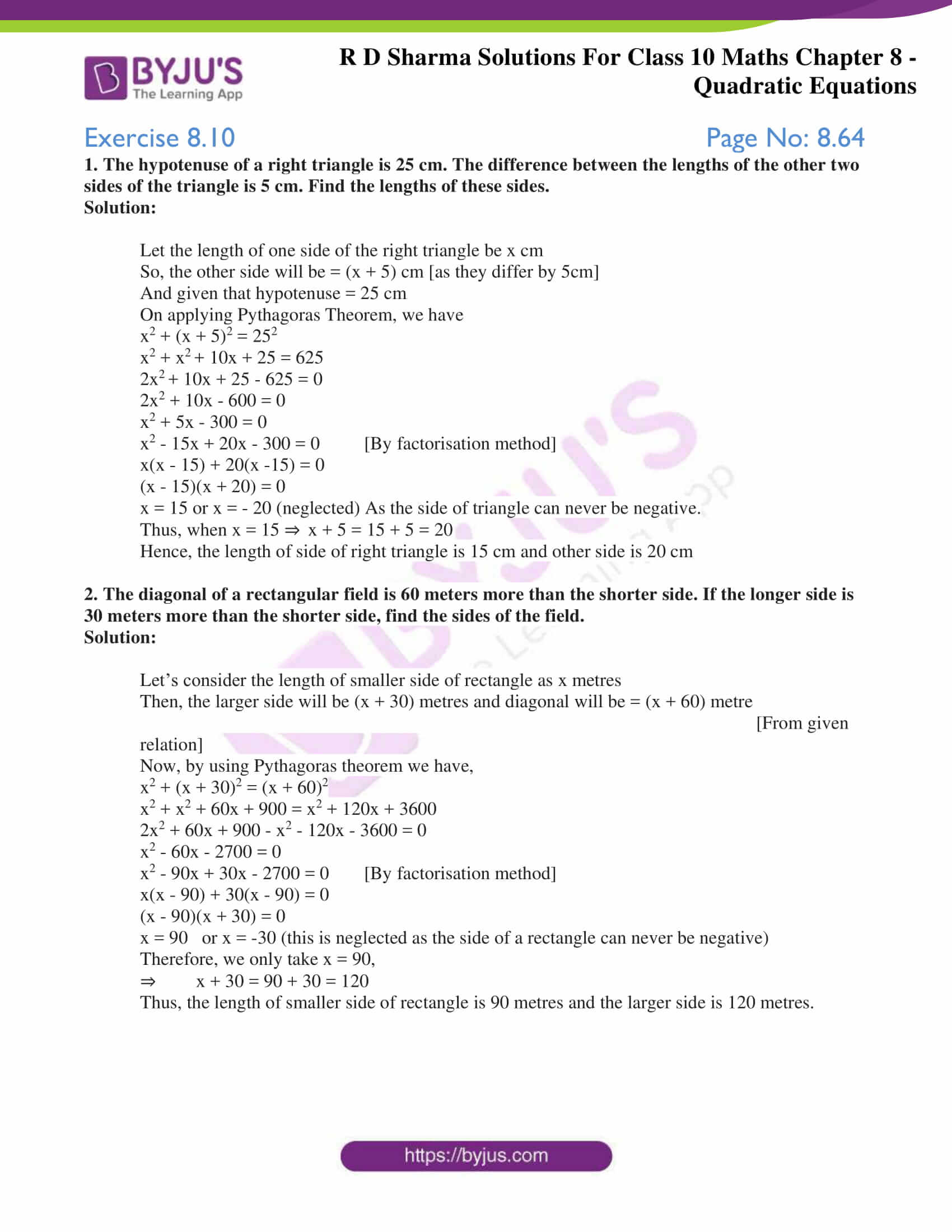# RD Sharma Solutions for Class 10 Maths Chapter 8 Quadratic Equations Exercise 8.10

Geometry is a vast topic of discussion. Application of quadratic equations for solving problems in geometry is discussed in this exercise. Students can access the RD Sharma Solutions Class 10 for getting a clear knowledge of solving problems pertaining to this chapter. Also, the students can make use of the RD Sharma Solutions for Class 10 Maths Chapter 8 Quadratic Equations Exercise 8.10 PDF given below as a reference when solving.### Access RD Sharma Solutions for Class 10 Maths Chapter 8 Quadratic Equations Exercise 8.10

1. The hypotenuse of a right triangle is 25 cm. The difference between the lengths of the other two sides of the triangle is 5 cm. Find the lengths of these sides.

Solution:

Let the length of one side of the right triangle be x cm

So, the other side will be = (x + 5) cm [as they differ by 5cm]

And given that hypotenuse = 25 cm

On applying Pythagoras Theorem, we have

x2 + (x + 5)2 = 252

x2 + x+ 10x + 25 = 625

2x+ 10x + 25 – 625 = 0

2x2 + 10x – 600 = 0

x2 + 5x – 300 = 0

x2 – 15x + 20x – 300 = 0 [By factorisation method]

x(x – 15) + 20(x -15) = 0

(x – 15)(x + 20) = 0

x = 15 or x = – 20 (neglected) As the side of triangle can never be negative.

Thus, when x = 15 ⇒ x + 5 = 15 + 5 = 20

Hence, the length of side of right triangle is 15 cm and other side is 20 cm

2. The diagonal of a rectangular field is 60 meters more than the shorter side. If the longer side is 30 meters more than the shorter side, find the sides of the field.

Solution:

Let’s consider the length of smaller side of rectangle as x metres

Then, the larger side will be (x + 30) metres and diagonal will be = (x + 60) metre

[From given relation]

Now, by using Pythagoras theorem we have,

x2 + (x + 30)2 = (x + 60)2

x2 + x2 + 60x + 900 = x2 + 120x + 3600

2x2 + 60x + 900 – x2 – 120x – 3600 = 0

x2 – 60x – 2700 = 0

x2 – 90x + 30x – 2700 = 0 [By factorisation method]

x(x – 90) + 30(x – 90) = 0

(x – 90)(x + 30) = 0

x = 90   or x = -30 (this is neglected as the side of a rectangle can never be negative)

Therefore, we only take x = 90,

⇒ x + 30 = 90 + 30 = 120

Thus, the length of smaller side of rectangle is 90 metres and the larger side is 120 metres.